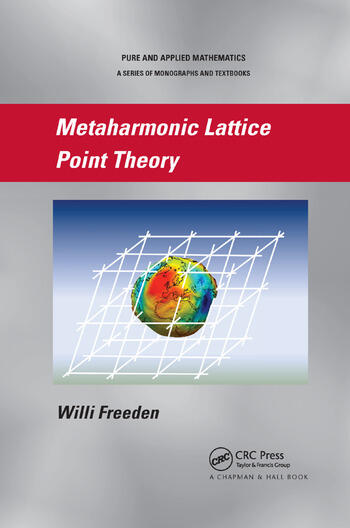# Metaharmonic Lattice Point Theory

## 1st Edition

Willi Freeden

Chapman and Hall/CRC
Published September 18, 2018
Reference - 472 Pages - 35 B/W Illustrations
ISBN 9781138382107 - CAT# K398530
Series: Chapman & Hall Pure and Applied Mathematics

For Instructors Request Inspection Copy

USD\$73.95

FREE Standard Shipping!

## Preview

### Summary

Metaharmonic Lattice Point Theory covers interrelated methods and tools of spherically oriented geomathematics and periodically reflected analytic number theory. The book establishes multi-dimensional Euler and Poisson summation formulas corresponding to elliptic operators for the adaptive determination and calculation of formulas and identities of weighted lattice point numbers, in particular the non-uniform distribution of lattice points.

The author explains how to obtain multi-dimensional generalizations of the Euler summation formula by interpreting classical Bernoulli polynomials as Green’s functions and linking them to Zeta and Theta functions. To generate multi-dimensional Euler summation formulas on arbitrary lattices, the Helmholtz wave equation must be converted into an associated integral equation using Green’s functions as bridging tools. After doing this, the weighted sums of functional values for a prescribed system of lattice points can be compared with the corresponding integral over the function.

Exploring special function systems of Laplace and Helmholtz equations, this book focuses on the analytic theory of numbers in Euclidean spaces based on methods and procedures of mathematical physics. It shows how these fundamental techniques are used in geomathematical research areas, including gravitation, magnetics, and geothermal.

#### Instructors

We provide complimentary e-inspection copies of primary textbooks to instructors considering our books for course adoption.